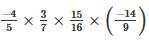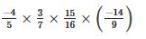Courses

# Test: Rational Numbers - 1

## 10 Questions MCQ Test Mathematics (Maths) Class 7 | Test: Rational Numbers - 1

Description
This mock test of Test: Rational Numbers - 1 for Class 7 helps you for every Class 7 entrance exam. This contains 10 Multiple Choice Questions for Class 7 Test: Rational Numbers - 1 (mcq) to study with solutions a complete question bank. The solved questions answers in this Test: Rational Numbers - 1 quiz give you a good mix of easy questions and tough questions. Class 7 students definitely take this Test: Rational Numbers - 1 exercise for a better result in the exam. You can find other Test: Rational Numbers - 1 extra questions, long questions & short questions for Class 7 on EduRev as well by searching above.
QUESTION: 1

### Associative property is not followed in _____

Solution:

In general associative properties also holds true addition as well as multiplication for all four options as well as but not true for subtraction as well as division.

If we add zero in the natural number then we also get set of numbers which is called as the whole number.

QUESTION: 2

### ____ is the identity for the addition of rational numbers.

Solution:

Additive identity means that to any number if this identity is added, the number remains the same. So additive identity is 0 which means 0 added to any no. does not affect it.

QUESTION: 3

### ____ is the multiplicative identity for rational numbers.

Solution:

1 is the multiplicative identity which means that identity of the number remains intact if it is multiplied by 1

QUESTION: 4

Which of the following is the reciprocal of the reciprocal of a rational number?

Solution:
QUESTION: 5

The product of two numbers is -20/9. If one of the numbers is 4, find the other.

Solution:

We have x * 4 = -20/9
x = -20 / 9* 4= -5/9

QUESTION: 6

The numbers ________ and ________ are their own reciprocals.

Solution:
QUESTION: 7

The reciprocal of – 5 is ________

Solution:
QUESTION: 8

Reciprocal of 1/x, where x ≠ 0 is ________.

Solution:

Reciprocal is the inverse of the number, meaning, the numerator becomes denominator and denominator becomes numerator.so 1/x become x/1

QUESTION: 9

The product of two rational numbers is always a _____.

Solution:
QUESTION: 10

simplify :Solution:⇒ -1 x 1 x 3/4 x -2/3

⇒ -1 x -1/2

⇒ 1/2